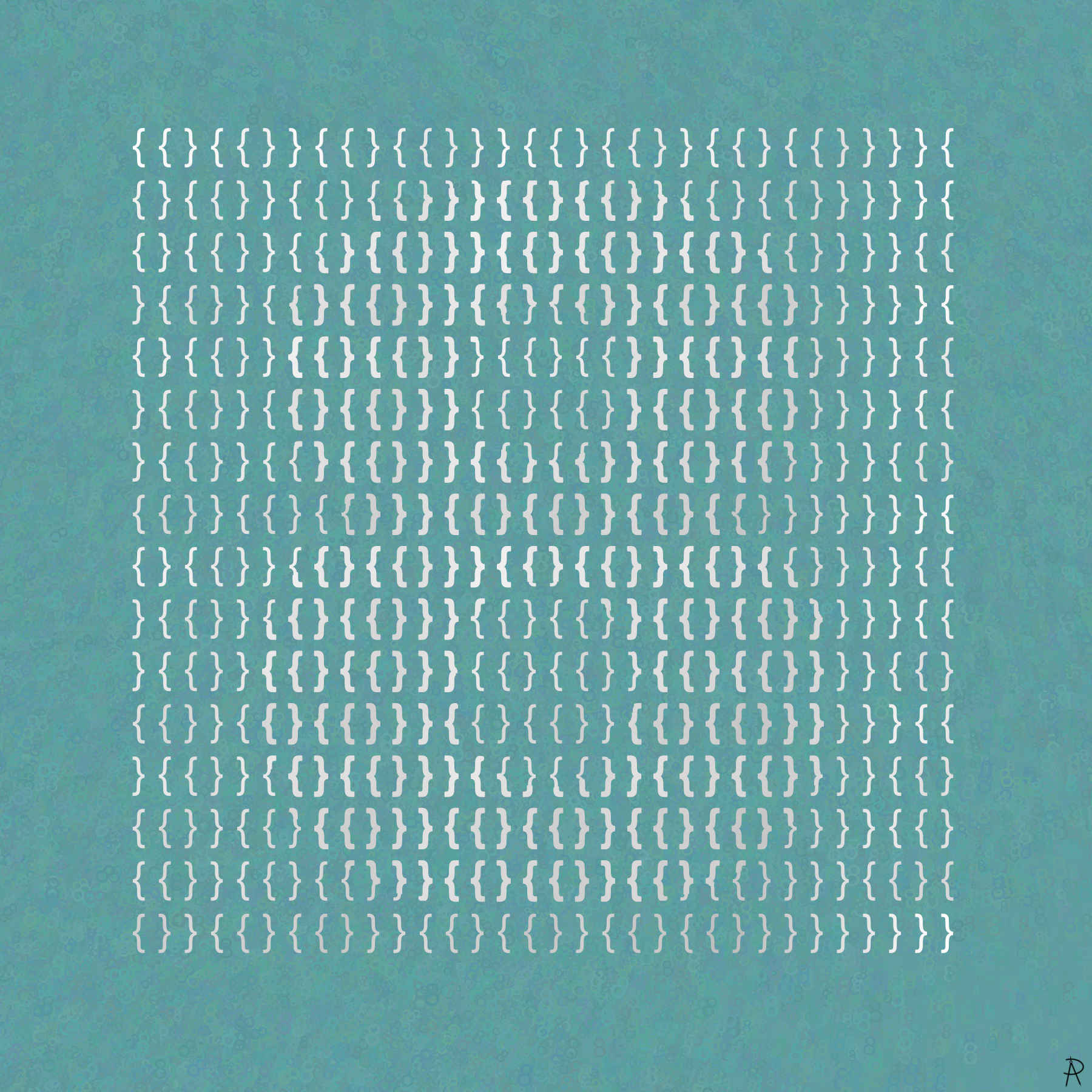Braces Of Eight Digital Print 2021

The number eight shown as a set-theoretic finite ordinal number using the construction of the non-negative integers described by John von Neumann around 1923.

### Background and Inspiration

How does one define the concept of number? What is the meaning of a number such as eight? One way to do this is from the perspective of a set.

In this set-theoretic finite ordinal number representation, zero is represented by the empty set: $$\{\}$$. Starting with $$0 = \{\}$$, we can define the integers recursively with the use of a successor function, $$s(n)$$, defined as follows: $s(n) = n + 1 = n \cup \{n\}.$ The successor of 0, $$s(0)$$, is 1: $1 = s(0) = 0 \cup \{0\} = \{\} \cup \{\{\}\} = \{\{\}\}.$ The successor of 1, $$s(1)$$, is 2: $2 = s(1) = 1 \cup \{1\} = \{\{\}\} \cup \{\{\{\}\}\} = \{ \{\}, \{\{\}\}\}.$ The successor of 2, $$s(2)$$, is 3: \begin{aligned} 3 = s(2) = 2 \cup \{2\} &= \{ \{\}, \{\{\}\}\} \cup \{\{ \{\}, \{\{\}\}\}\} \\ &= \{\{\},\{\{\}\},\{\{\},\{\{\}\}\}\}.\\ \end{aligned} The successor of 3, $$s(3)$$, is 4: \begin{aligned} 4 &= s(3) \\ &= 3 \cup \{3\} \\ &= \{\{\},\{\{\}\},\{\{\},\{\{\}\}\}\} \cup \{\{\{\},\{\{\}\}, \{\{\},\{\{\}\}\}\}\} \\ &= \{\{\}, \{\{\}\}, \{\{\},\{\{\}\}\},\{\{\},\{\{\}\},\{\{\},\{\{\}\}\}\}\}.\\ \end{aligned} In general, the number $$n$$ is the successor of $$n-1$$ and is the set containing the numbers $$0$$ through $$n-1$$: \begin{aligned} s(n-1) &= (n-1) \cup \{(n-1)\}\\ &= \underbrace{(n-2) \cup \{(n-2)\}}_{(n-1)} \cup \{(n-1)\}\\ &= \underbrace{(n-3) \cup \{(n-3)\}}_{(n-2)} \cup \{(n-2)\} \cup \{(n-1)\}\\ &= \cdots\\ &= 0 \cup \{(0)\} \cup \{(1)\} \cup \{(2)\} \cup \cdots \cup \{(n-1)\} \\ &= \{\} \cup \{0, 1, 2, \ldots, n-1\}.\\ &= \{0, 1, 2, \ldots, n-1\}.\\ \end{aligned}

A nice feature of this representation is that the number of elements of each set (its cardinality) is just the number itself. For example, the set representing 8 is $$\{0,1,2,3,4,5,6,7,\}$$, which has 8 elements. Note these elements are generally sets containing other elements that are sets.

An interesting property of this construction is that $$a < b$$ can be defined using set operations. Let $$a$$ and $$b$$ be two numbers and $$A$$ and $$B$$ be the two sets representing them respectively. Then $$a < b$$ if and only if $$A \subset B$$, where is $$A$$ is a proper subset of $$B$$.

Note the commas in the representation are extraneous, so juxtaposition is sufficient in expressing the ordinals. The number of $$\{$$ symbols needed to represent $$n$$ is $$2^n$$. Similarly, $$2^n$$ $$\}$$ symbols are needed, resulting in a total of $$2^{n+1}$$ required symbols. Thus 512 total symbols are needed to represent 8.

The artwork Braces of Eight uses just the symbols $$\{$$ and $$\}$$ to represent eight. The thickness of the symbols are varied to show the numeral 8. The background texture is made from randomly sized, colored, and placed 8's as shown below.